# Yield Stress Calculation Methods

The traditional method for determining yield stress on a rotational rheometer or viscometer was by fitting models to the measured rheograms and extrapolating to zero shear rate. This article discusses various yield stress calculation methods.

## Models for Yield Stress Calculation

The Bingham model is simple and is used to describe the behavior of concentrated suspensions of solid particles in Newtonian liquids. The Bingham model can be written mathematically as: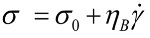Where σ0 is the yield stress and ηB is the Bingham viscosity or plastic viscosity. It should be noted that the Bingham viscosity is not a real viscosity value; it just describes the slope of the Newtonian portion of the curve.

The Casson model is an alternative model to the Bingham model. This model has all components in the Bingham equation raised to the power of 0.5 and has a more gradual transition between the yield and Newtonian regions. The Casson equation can be written as,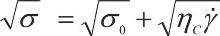where σ0 is the yield stress and ηC is the Casson viscosity, which relates to the high shear rate viscosity. The Herschel-Bulkley model describes non-Newtonian behavior after yielding and is basically a power law model with a yield stress term.

Herschel-Bulkley equation is written as follows;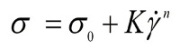where K is the consistency and n is the shear thinning index. The latter term describes the degree to which a material is shear thinning (n < 1) or shear thickening (n > 1).

Stress-shear rate curves for a Herschel-Bulkley and Bingham type fluid are shown in Figure 1. Note these are presented on linear scaling but will have different profiles when displayed logarithmically, which is how such curves are usually represented.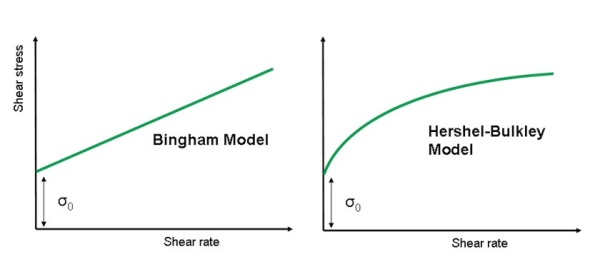Figure 1. Illustration of Bingham and Herschel-Bulkley model fits using linear scaling.

In order to determine which model is most appropriate it is necessary to measure the steady shear stress over a range of shear rates and fit each model to the data.

There are additional models that can be used to estimate the yield stress, or more appropriately, the critical shear stress for materials having a zero shear viscosity. These additional models are modified versions of the Ellis and Cross models for viscosity versus shear stress and viscosity versus shear rate data respectively.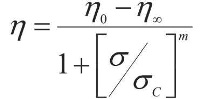where η is the viscosity, η0 is the zero shear viscosity, σ is the stress and σC is the critical shear stress. The critical shear stress is the stress at which the onset of non-linearity occurs and is essentially the asymptotic value of the shear stress at infinite viscosity assuming power law behavior as shown in Figure 2.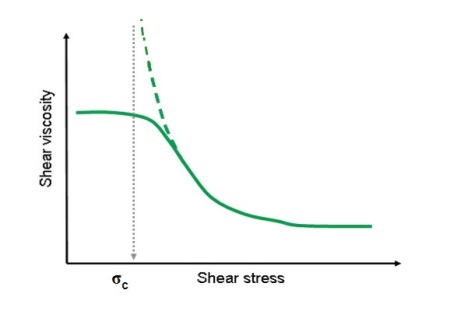Figure 2. Illustration showing an Ellis model fitted to the flow curve of a shear thinning liquid.

## Stress Ramp

A highly rapid and easy method for yield stress measurement on a stress controlled rheometer is to perform a shear stress ramp and determine the stress at which a viscosity peak is observed as shown in Figure 3.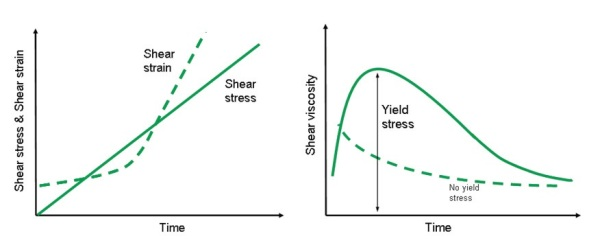Figure 3. Shear stress-strain curve (left) and corresponding viscosity-stress curve (right) for materials with and without a yield stress.

## Stress Growth

A constantly increasing stress is applied with a stress ramp test and the resultant strain rate or shear rate monitored with time. Conversely, a stress growth test involves application of a constantly increasing strain (constant shear rate) and monitoring the stress build-up with time.

The sample is subject to work hardening resulting from elastic elements being stretched in the shear field below its critical strain.

## Oscillation Amplitude Sweep

This test involves applying an increasing oscillatory stress or strain and monitoring the corresponding changes in the elastic modulus (G'), or the elastic stress (C) with increasing amplitude. There are different ways of interpreting yield stress from an amplitude sweep, as shown diagrammatically in Figure 4.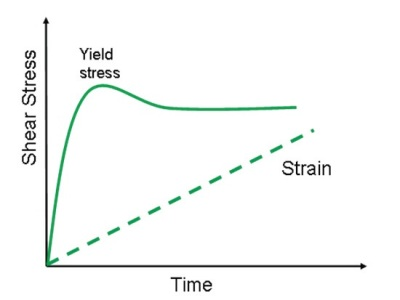Figure 4. Illustration showing points commonly used to determine the yield stress and strain from an oscillation amplitude sweep.

A more recent method for determining yield stress by means of oscillation testing involves measuring the elastic stress component (C), which is associated with the elastic modulus (G'), as a function of strain amplitude. The yield stress is taken to be the peak value of the elastic stress, and the corresponding strain value the yield strain.

It is important to note that test frequency can influence the measured yield stress based on the relaxation behavior of the material under test.

## Multiple Creep

A multiple creep test is one of the most accurate methods for determining yield stress. This involves performing a series of creep tests using different applied stresses and looking for changes in the gradient of the compliance versus time curve.

Depending upon the nature of the material being tested, the response can be quite different, as shown in Figure 5.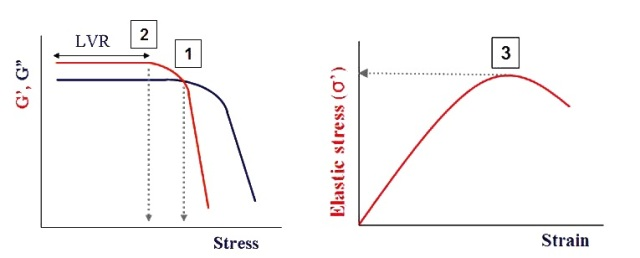Figure 5. Illustration showing the strain response to an applied stress.

The creep shear compliance (J) can be determined from the preset shear stress (CT) and the resulting deformation (y) through:

## Tangent Analysis

Tangent analysis is another common method for determining yield stress, which can be used in both oscillatory and steady shear techniques as shown in Figure 6. In oscillatory tests, if a single tangent is applied to the linear region of the curve then the yield stress is often taken as the stress at which the curve begins to deviate from this tangent.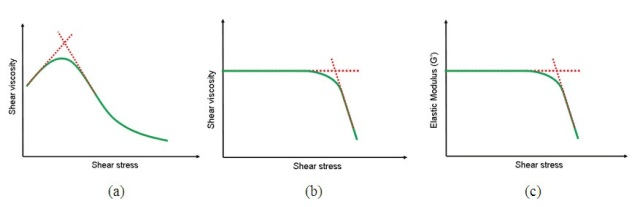Figure 6. Illustration showing yield stress/critical stress determination by tangent analysis using steady shear testing (a and b) and oscillation testing. (c)This information has been sourced, reviewed and adapted from materials provided by Malvern Panalytical.

## Citations

• APA

Malvern Panalytical. (2019, November 04). Yield Stress Calculation Methods. AZoM. Retrieved on October 21, 2020 from https://www.azom.com/article.aspx?ArticleID=9929.

• MLA

Malvern Panalytical. "Yield Stress Calculation Methods". AZoM. 21 October 2020. <https://www.azom.com/article.aspx?ArticleID=9929>.

• Chicago

Malvern Panalytical. "Yield Stress Calculation Methods". AZoM. https://www.azom.com/article.aspx?ArticleID=9929. (accessed October 21, 2020).

• Harvard

Malvern Panalytical. 2019. Yield Stress Calculation Methods. AZoM, viewed 21 October 2020, https://www.azom.com/article.aspx?ArticleID=9929.